American Journal of Modern Physics
Volume 4, Issue 5-1, October 2015, Pages: 35-37

Santilli Isomathematics for Generalizing Modern Mathematics

Chun-Xuan Jiang

Institute for Basic Research, Beijing, P. R. China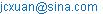Chun-Xuan Jiang. Santilli Isomathematics for Generalizing Modern Mathematics. American Journal of Modern Physics. Special Issue: Issue I: Foundations of Hadronic Mathematics. Vol. 4, No. 5-1, 2015, pp. 35-37. doi: 10.11648/j.ajmp.s.2015040501.14

Abstract: The establishment of isomathematics, as proposed by R. M. Santilli thirty years ago in the USA, and contributed to by Jiang Chun-Xuan in China during the past 12 years, is significant and has changed modern mathematics. At present, the primary teaching of mathematics is based on the simple operations of addition, subtraction, multiplication and division; a middle level teaching ofmathematics takes these four operations to a higher level, while the university teaching of mathematics extends them to an even higher level. These four arithmetic operations form the foundation of modern mathematics. Santilli isomathematics is a generalisation of these four fundamental operations and heralds a great revolution in mathematics. HIn this paper, we study the four generalized arithmetic operations of isoaddition, isosubtraction, isomultiplication and isodivision at the primary level of isomathematics. The material introduced here should be readily understandable by middle school pupils and university students.Santilli’s isomathematics  ßßis based on a generalisation of modern mathematics. Isomultiplication is defined by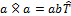, isodivision by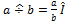, where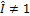is called an isounit;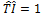, where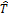is the inverse of the isounit. If addition and subtraction remain unchanged,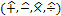are the four arithmetic operations in Santilli’s isomathematics[1-5]. Isoaddition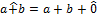and isosubtraction, where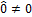is called the isozero, together with the operations of isomultiplication and isodivision introduced above, form the four arithmetic operationsin Santilli-Jiang isomathematics. Santilli  suggests isomathematics based on a generalisation of multiplication ×, division ÷, and the multiplicative unit 1 of modern mathematics. It is an epoch making suggestion. From modern mathematics, the foundations of Santilli’s isomathematics will be established.

Keywords: Santilli-Jiang Math, Isomultiplication, Isodivision, Isoaddition and Isosubtraction

1. Division and Multiplication in Modern Mathematics

Suppose that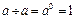(1)

where 1 is the multiplicative unit and 0 is exponent zero.

From (1), division ÷ and multiplication × are defined by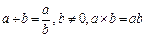(2)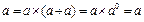(3)

Now consider the multiplicative unit 1,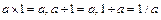(4)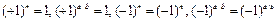(5)

Addition +, subtraction -, multiplication ×, and division ÷ are the four operations forming the foundation of modern mathematics. The modern mathematics is generalised to establish the foundations of Santilli-Jiang isomathematics.

2. Isodivision and Isomultiplication in Santilli’s Isomathematics

Isodivisionand isomultiplication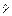[1 – 5], which are generalisations of the division ÷ and multiplication × of modern mathematics, are now defined.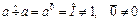(6)

where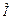is called the isounit and is a generalisation of the multiplicative unit 1 and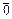is the isoexponent zero which is a generalisation of the exponent zero 0. Then,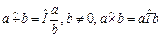(7)

It is seen that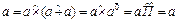(8)

from which it follows that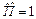(9)

where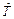is the inverse of the isounit.

The isounithas the following properties[5,p93-95,isoexponents]: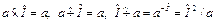(10)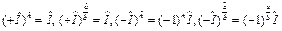(11)

With addition and subtraction unchanged,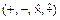are the four arithmetic operations in Santilli’s isomathematics and these form the foundations of Santilli isomathematics. When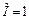, the operations revert to being those of the modern mathematics.

3. Addition and Subtraction in Modern Mathematics

These are defined by

x = a + b and y = a – b            (12)

a + a – a = a                (13)

a – a = 0                    (14)

Isoaddition and isosubtraction may be established using these results.

4. Isoaddition and Isosubtraction in Santilli-Jiang Isomathematics

Isoaddition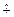and isosubtraction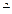are defined by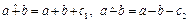(15)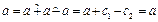(16)

Then, from (16), it follows that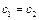(17)

Suppose that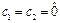, where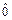is called the isozero which is a generalisation of the zero 0 of addition and subtraction. Hence,(18)

When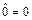, these equations are the usual laws of addition and subtraction of modern mathematics.

From the above results, the foundations of Santilli-Jiang isomathematics are readily established: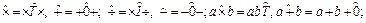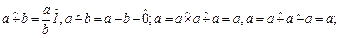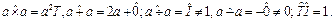(19)

Here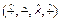are the four arithmetic operations in Santilli-Jiang isomathematics.

Remark: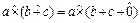From the left-hand side, it follows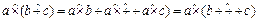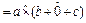,

where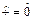is a number also.

Again,From the left-hand side of this relation, it is seen that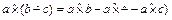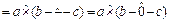,

whereis a number also.

The distributive laws are satisfied and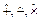,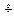are numbers.

This Santilli-Jiang isomathematics therefore, provides a new mathematical tool for studying the mathematical problems of the 21st century and helping in the understanding the mysteries of our universe.

5. An Illustrative Example

Consider the algebraic equation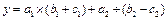(20)

(20) may represent a mathematical system, physical system, biological system,cryptogram system, IT system, or some other system. It may be written as the isomathematical equation(21)

If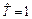andthen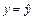.

Let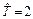and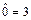. From (21) we have the isomathematical subequation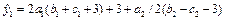(22)

Let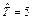and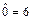. From (21) we have the isomathematical subequation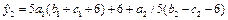(23)

Let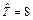and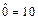. From (21) we have the isomathematical subequation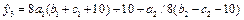(24)

Therefore, (21) has infinitely many isomathematical subequations. Using (21) – (24),and, the stability and optimum structure of the algebraic equation (20) may be studied.

References

1. R. M. Santilli, Rendiconti Circolo Matematico Palermo, Suppl. Vol. 42, 7-82 (1996),\\http://www.santilli-foundation.org/docs/Santilli-37.pdf
2. Chun-Xuan Jiang, (1998), Foundations of Santilli’s isonumber theory, Part I: Isonumber theory of the first kind; Algebras, Groups and Geometries, 15, 351-393.
3. Chun-Xuan Jiang, (1998), Foundations of Santilli’s isonumber theory, Part II: Isonumber theory of the second kind; Algebras Groups and Geometries, 15, 509-544.
4. Chun-Xuan Jiang, (1999), Foundations of Santilli’s isonumber theory, in: Fundamental open problems in sciences at the end of the millennium, T. Gill, K. Liu and E. Trell (Eds), Hadronic Press, USA, 105-139.
5. Chun-Xuan Jiang, (2002), Foundations of Santilli’s isonumber theory, with applications to new cryptograms, Fermat’s theorem and Goldbach’s conjecture, International Academic Press, America- Europe- Asia.MR2004c:11001.(also available in the pdf file http: // www. i-b-r. org/docs/jiang.pdf)(http://vixra.org/pdf/1303.0088v1.pdf))
6. Chun-Xuan Jiang,(2008),Santilli-Jiang isomathematics for changing modern mathematics,middle school mathematics(Chinese),Dec.46-48

 Contents 1. 2. 3. 4. 5.
Article ToolsAbstractPDF(153K)